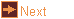# polygon - polygon based polyhedra

 Usage    |    Examples    |    Notes

## Usage

```

Usage: polygon [options] type num_sides

Make polyhedra based on polygons. The polyhedron type and subtype may be
given by (partial) name or number
1. prism (angle: polygons twist, forming an antiprism)
subtypes: 1. antiprism, from triangulating side faces
2. trapezohedron, with this antiprism-ised belt
3. crown (-A integer to specify a second polygon step)
2. antiprism (angle: polygons twist)
subtypes: 1. trapezohedron, with this antiprism belt
2. antihermaphrodite, with this antiprism base
3. scalenohedron (-L for apex height)
4. subdivided_scalenohedron (-L for apex height)
5. crown (-A integer to specify a second polygon step)
3. pyramid (angle: base separates, polygons twist to antihermaphrodite)
subtypes: 1. antihermaphrodite, with this antiprism base
2. elongated (-L for prism height)
3. gyroelongated (-L for antiprism height)
4. dipyramid (angle: base separates, polygons twist to trapezohedron)
subtypes: 1. trapezohedron, with this pyramid apex
2. elongated (-L for prism height)
3. gyroelongated (-L for antiprism height)
4. dipyramid_scalenohedron (-R for alternate vertex radius)
5. cupola
subtypes: 1. elongated (-L for prism height)
2. gyroelongated (-L for antiprism height)
3. cupoloid
6. orthobicupola
subtypes: 1. elongated (-L for prism height)
2. gyroelongated (-L for antiprism height)
7. gyrobicupola
subtypes: 1. elongated (-L for prism height)
2. gyroelongated (-L for antiprism height)
8. snub-antiprism
subtypes: 1. inverted, triangle band inverted
9. dihedron
subtypes: 1. polygon
10. crown polyhedron (either -A integer to specify a second polygon step,
or -a angle to specify a twist

num_sides is a number (N) optionally followed by / and a second
number (N/D). N is the number of vertices spaced equally on a
circle, and each vertex is joined to the Dth (default: 1) vertex
moving around the circle.

Options
-h,--help this help message (run 'off_util -H help' for general help)
--version version information
-s <subt> a number or name (see type list above) indicting a subtype
or modification of a polyhedron
-e <len>  length of polygon edges (default: 1)
-E <len>  length of non-polygon edges (default: calculate from -l)
-l <hgt>  height of upper vertices above base polygon
-L <hgt>  a second height or length value
-a <ang>  twist angle (degrees)
-A <ang>  a second twist angle (degrees)
-o <file> write output to file (default: write to standard output)

```

## Examples

A cube with edges of 2 units
```polygon -e 2 -l 2 pri 4 | antiview
```

A hexagonal based pyramid
```polygon pyr 6 | antiview
```

A uniform pentagrammic antiprism
```polygon -e 1 -E 1 ant 5/2 | antiview
```

A uniform pentagrammic crossed antiprism
```polygon -e 1 -E 1 ant 5/3 | antiview
```

A uniform crossed gyroelongated pentagrammic dipyramid
```polygon -e 1 -E 1 -s gyro dip 5/3 | antiview
```

A hexagonal trapezohedron
```polygon -s trap dip 6  | antiview
```

A twisted hexagonal trapezohedron
```polygon -s trap -a 45 dip 6  | antiview
```

## Notes